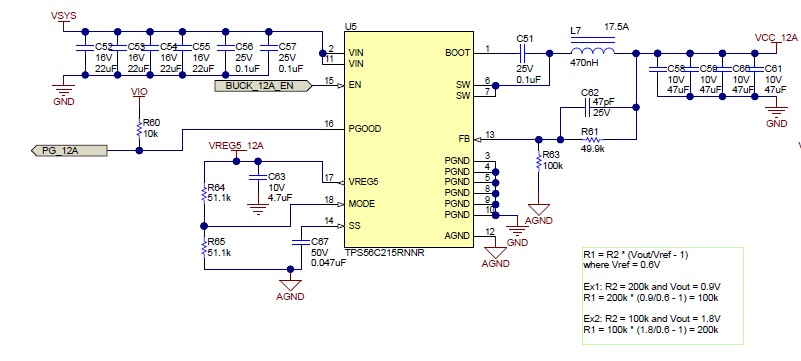If you have a related question, please click the "Ask a related question" button in the top right corner. The newly created question will be automatically linked to this question.

• Resolved

# TPS56C215: Supply 0.85V to Zynq FPGA

Part Number: TPS56C215

HI.

I used TPS56C215 for supply 0.85v to Xilinx FPGA Zynq Ultrascle+ MPSoC.

I made a supply circuit by referencing "Xilinx-Zynq-US+ Scalable PMIC Power Design"I want to confirm the question

1. To obtain 0.85V for VCC_12A,

R63 = R62 * (Vout/Vref -1)

where  Vout = 0.85V, Vref = 0.6V, R62= 100kΩ

R63 = 100k * (0.85/0.6 -1 ) ≒41.6KΩ

Is that right ?

2.  Is  Output Capacitance(C58-61) and Inductance (L7) for Vout 0.85V is the same with each value in  reference circuit ?

3.  May I use VREG5  for VIO which connects PGOOD signal in reference circuit ?

4. For EN signal (BUCK_12A_EN), can I connect  to the center tap of the resistor divder between  VSYS(5V) and AGND to get over 1.2V

• Hi,

1, For FB resistor, if the Vout is 0.85V, recommend the upper resistor value 10K, and lower resistor value 24K.

2, If the input Vsys is only 5V and I see the mode you select is 1.2M Frequency, if so, the inductor can be lower to 0.33uH or 0.22uH.

3, OK

4, OK

Yuchang

• In reply to Yuchang Zhang: